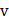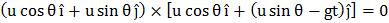# A particle is projected from the ground at an anglewith the horizontal with an initial speed. At what time after which velocity vector of projectile is perpendicular to the initial velocity? [in second]

## Question ID - 153864 :- A particle is projected from the ground at an anglewith the horizontal with an initial speed. At what time after which velocity vector of projectile is perpendicular to the initial velocity? [in second]

3537

(4)

Initial velocityVelocity after timeisSinceandare perpendicular to each otherNext Question :
 Two particleandmove with constant velocitiesandalong two mutually perpendicular straight lines towards the intersection point. At moment, the particles were located at distancesandfrom, respectively. Find the time when they are nearest and also this shortest distance (nearest integer)